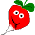## Monday, March 16, 2009

### String and ASCII Code Conversion in VB 2005

We have written a customized class for various conversion between ASCII code and string that we need to use occasionally. The source code for the class can be downloaded A Visual Basic class to convert between hexadecimal, ascii, and text string on GitHub.
'Author: Yan Naing Aye
'WebSite: http://cool-emerald.blogspot.sg/
'Updated: 2009 April 24
'-----------------------------------------------------------------------------
Public Class ClsMyStr
Public Shared Function AsAsciiEncodedStr(ByVal CharString As String) As String
Dim outS As String = ""
Dim temp As String = ""
Dim i As Integer = 0
For i = 0 To CharString.Length - 1
temp = "00" & Hex(Asc(CharString(i)))
temp = Right(temp, 2)
outS = outS & temp
Next i
Return outS
End Function
Public Shared Function AsSpacedAsciiEncodedStr(ByVal CharString As String) As String
Dim outS As String = ""
Dim temp As String = ""
Dim i As Integer = 0
For i = 0 To CharString.Length - 1
temp = "00" & Hex(Asc(CharString(i)))
temp = Right(temp, 2)
outS = outS & temp & " "
Next i
Return outS
End Function
Public Shared Function AsAsciiDecodedStr(ByVal AsciiEncodedStr As String) As String
Dim outS As String = ""
Dim i As Integer = 0
Dim l As Integer = AsciiEncodedStr.Length - 2

If (AsciiEncodedStr.Length Mod 2) <> 0 Then
l -= 1
End If
For i = 0 To l Step 2
outS = outS & Chr(Val("&H" & AsciiEncodedStr.Substring(i, 2)))
Next i
Return outS
End Function
Public Shared Function GetAsciiEncodedStr(ByVal RawAsciiEncodedStr As String) As String
Dim i As Integer = 0
Dim c As String
Dim cmd As String = ""

For i = 0 To RawAsciiEncodedStr.Length - 1
c = RawAsciiEncodedStr(i)
If (Asc(c) >= &H30) AndAlso (Asc(c) <= &H39) Then
cmd = cmd & c
ElseIf (Asc(c) >= &H41) AndAlso (Asc(c) <= &H5A) Then
cmd = cmd & c
ElseIf (Asc(c) >= &H61) AndAlso (Asc(c) <= &H7A) Then
cmd = cmd & Chr(Asc(c) - &H20) 'change to upper case
Else
'MessageBox.Show("Got invalid character.")
End If
Next i
If cmd.Length < 2 Then
cmd = "0" & cmd
End If
Return cmd
End Function
Public Shared Function DoubleQuote() As String
Return ControlChars.Quote
End Function
Public Shared Function Byte2Text(ByVal byteArray() As Byte) As String
Dim str As String = BitConverter.ToString(byteArray)
Return str
End Function
Public Shared Function Byte2Str(ByVal byteArray() As Byte) As String
'Dim str As String = System.Text.Encoding.ASCII.GetString(byteArray)
Dim str As String = ""
For i As Integer = 0 To UBound(byteArray)
str &= Chr(byteArray(i))
Next
Return str
End Function
Public Shared Function Str2Byte(ByVal str As String) As Byte()
'Dim ba() As Byte = System.Text.Encoding.ASCII.GetBytes(str)
Dim ba() As Byte
Try
ReDim ba(str.Length - 1)
For i As Integer = 0 To UBound(ba)
ba(i) = Asc(str(i))
Next
Catch ex As Exception
ReDim ba(0)
ba(0) = 0
End Try
Return ba
End Function
Public Shared Function GetSignedDecimalText(ByVal RawStr As String) As String
Dim i As Integer = 0
Dim c As String
Dim cmd As String = ""

For i = 0 To RawStr.Length - 1
c = RawStr(i)
If (Asc(c) >= &H30) AndAlso (Asc(c) <= &H39) Then
cmd = cmd & c
ElseIf (Asc(c) = &H2D) Then
If cmd.Length = 0 Then
cmd = cmd & c
End If
Else
'MessageBox.Show("Got invalid character.")
End If
Next i
Return cmd
End Function
End Class



1.This was very helpful for me--thank you for sharing it!

2.Sir,how many union structures can I use in a firmware c program?

3.Hi Thida, it should be limited by the amount of physical memory in your microcontroller, shouldn't it?

Comments are moderated and don't be surprised if your comment does not appear promptly.